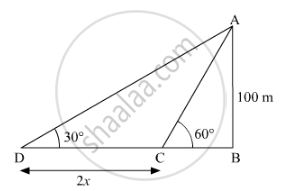# A Man in a Boat Rowing Away from a Lighthouse 100 M High Takes 2 Minutes to Change the Angle of Elevation of the Top of the Lighthouse from 60° to 30°. - Mathematics

Sum

A man in a boat rowing away from a lighthouse 100 m high takes 2 minutes to change the angle of elevation of the top of the lighthouse from 60° to 30°.
Find the speed of the boat in metres per minute. [Use sqrt(3 = 1.732]Use 3=1.732">

#### SolutionAB is a lighthouse of height 100m.
Let the speed of the boat be x metres per minute.
And CD is the distance which man travelled to change the angle of elevation.

So, CD = 2x   ...[∵ Distance = Speed x Time]

tan (60°) = ("AB")/("BC")

sqrt(3) = (100)/("BC")

⇒ "BC" = (100)/(sqrt(3)

tan (30°) = ("AB")/("BD")

(1)/sqrt(3) = (100)/("BD")

"BD" = 100 sqrt(3)
"CD"= "BD" - "BC"

2"x" = 100sqrt(3) - (100)/sqrt(3)

2"x" = (300-100)/sqrt(3)

⇒ "x" = (200)/(2sqrt(3)

⇒ "x" = (100)/(sqrt3)

Using sqrt(3) = 1.73

"x" = (100)/(1.73) ≈ 57.80

Hence, the speed of the boat is 57.80">57.8057.80 metres per minute.

Concept: Heights and Distances
Is there an error in this question or solution?